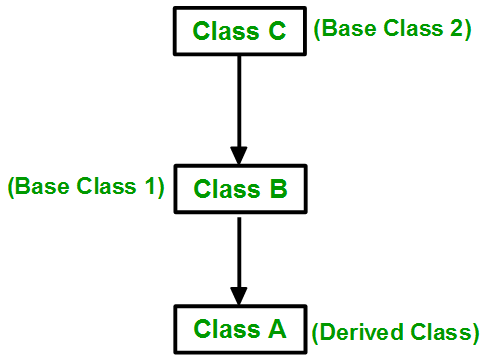GFG App
Open AppBrowser
Continue

# Constructor in Multilevel Inheritance in C++

Constructor is a class member function with the same name as the class. The main job of the constructor is to allocate memory for class objects. Constructor is automatically called when the object is created.

### Multilevel Inheritance

Derivation of a class from another derived class is called Multilevel Inheritance. Class A is the base class for the derived class B, which in turn serves as a base class for the derived class C. Class B provides a link for the inheritance between A and C and is known as an intermediate base class. The chain A, B, C is known as the inheritance path.Constructor in Multilevel Inheritance

Example 1: Below is the C++ program to show the concept of constructor in multilevel Inheritance.

## C++

 `// C++ program to implement ` `// constructor in multilevel  ` `// Inheritance ` `#include ` `using` `namespace` `std; ` ` `  `// Base class ` `class` `A ` `{ ` `    ``public``: ` `        ``A() ` `        ``{ ` `            ``cout << ``"Base class A constructor \n"``; ` `        ``} ` `}; ` ` `  `// Derived class B ` `class` `B: ``public` `A ` `{ ` `    ``public``: ` `        ``B() ` `        ``{ ` `            ``cout << ``"Class B constructor \n"``; ` `        ``} ` `}; ` ` `  `// Derived class C ` `class` `C: ``public` `B ` `{ ` `    ``public``: ` `        ``C() ` `        ``{ ` `            ``cout << ``"Class C constructor \n"``; ` `             `  `        ``} ` `}; ` ` `  `// Driver code ` `int` `main() ` `{ ` `    ``C obj; ` `    ``return` `0; ` `}`

Output

```Base class A constructor
Class B constructor
Class C constructor ```

Example 2: Below is the C++ program to implement multilevel inheritance.

## C++

 `// C++ program to implement  ` `// multilevel inheritance ` `#include ` `using` `namespace` `std; ` ` `  `// Base class A ` `class` `A ` `{ ` `    ``public``: ` `        ``A() ` `        ``{ ` `            ``int` `a = 5, b = 6, c; ` `            ``c = a + b; ` `            ``cout << ``"Sum is:"` `<<  ` `                     ``c << endl; ` `        ``} ` `}; ` ` `  `// Class B ` `class` `B: ``public` `A ` `{ ` `    ``public``: ` `        ``B() ` `        ``{ ` `            ``int` `d = 50,e = 35, f; ` `            ``f = d - e; ` `            ``cout << ``"Difference is:"` `<< ` `                     ``f << endl; ` `        ``} ` `}; ` ` `  `// Derived class C ` `class` `C: ``public` `B ` `{ ` `    ``public``: ` `        ``C() ` `        ``{ ` `            ``int` `g = 10, h = 20, i; ` `            ``i = g * h; ` `            ``cout << ``"Product is:"` `<< ` `                     ``i << endl;             ` `        ``} ` `}; ` ` `  `// Driver code ` `int` `main() ` `{ ` `    ``C obj; ` `    ``return` `0; ` `}`

Output

```Sum is:11
Difference is:15
Product is:200```

My Personal Notes arrow_drop_up